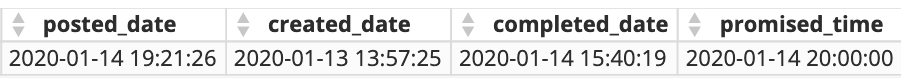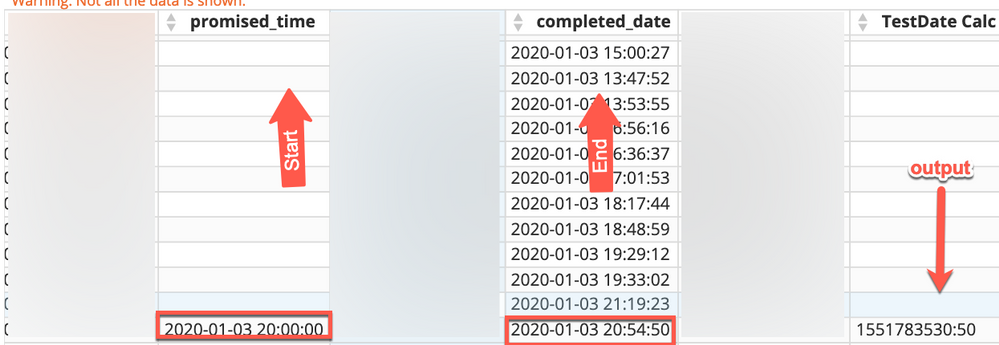# Date Calculations - minutes and seconds

Hi, Experts,

I am working on trying to find out how to calculate the time difference between dates.Here is what I am after:

I would like to see the difference lets pick two dates start (created_date) - end (posted_date). I would like the output to show the time in minutes:seconds or minutes.seconds of the lapse between the time. If you also apply that same formula that will be generated to another set of dates, lets say start (completed_date) - end (promised_time) will the output be more or less the same or will the calculation need to be different for the latter?

-Isaiah

• @jaeW_at_Onyx - Yes. UNIX_TIMESTAMP will convert the date to the number of seconds since 1970-01-01 00:00:00 so subtracting those two together will get you the total elapsed seconds between two date times.

If you're wanting to display it as MInutes : Seconds you'd need to do some additional math.

The following beast modes return the date parts in that elapsed time. They're utilizing CURRENT_TIMESTAMP and a `Date` field to show elapsed time from now. If you want to use two different fields replace CURRENT_TIMESTAMP with your END timestamp and `Date` with your START timestamp.

Days:

`ROU D((UNIX_TIMESTAMP(CURRENT_TIMESTAMP()) - UNIX_TIMESTAMP(`Date`) - MOD(UNIX_TIMESTAMP(CURRENT_TIMESTAMP()) - UNIX_TIMESTAMP(`Date`), 86400)) / 86400)`

Hours:

`ROUND((MOD(UNIX_TIMESTAMP(CURRENT_TIMESTAMP()) - UNIX_TIMESTAMP(`Date`), 86400) - MOD(MOD(UNIX_TIMESTAMP(CURRENT_TIMESTAMP()) - UNIX_TIMESTAMP(`Date`), 86400), 3600)) / 3600)`

Minutes:

`ROUND((MOD(UNIX_TIMESTAMP(CURRENT_TIMESTAMP()) - UNIX_TIMESTAMP(`Date`), 3600) - MOD(MOD(UNIX_TIMESTAMP(CURRENT_TIMESTAMP()) - UNIX_TIMESTAMP(`Date`), 3600), 60)) / 60)`

Seconds:

`ROUND(MOD(UNIX_TIMESTAMP(CURRENT_TIMESTAMP()) - UNIX_TIMESTAMP(`Date`), 60) - 0.5) -- Whole elapsed seconds`

Finally, bringing it all together:

`CONCAT(  ROUND((UNIX_TIMESTAMP(CURRENT_TIMESTAMP()) - UNIX_TIMESTAMP(`Date`) - MOD(UNIX_TIMESTAMP(CURRENT_TIMESTAMP()) - UNIX_TIMESTAMP(`Date`), 86400)) / 86400), 'd ',  ROUND((MOD(UNIX_TIMESTAMP(CURRENT_TIMESTAMP()) - UNIX_TIMESTAMP(`Date`), 86400) - MOD(MOD(UNIX_TIMESTAMP(CURRENT_TIMESTAMP()) - UNIX_TIMESTAMP(`Date`), 86400), 3600)) / 3600), ':',  ROUND((MOD(UNIX_TIMESTAMP(CURRENT_TIMESTAMP()) - UNIX_TIMESTAMP(`Date`), 3600) - MOD(MOD(UNIX_TIMESTAMP(CURRENT_TIMESTAMP()) - UNIX_TIMESTAMP(`Date`), 3600), 60)) / 60), ':',  ROUND(MOD(UNIX_TIMESTAMP(CURRENT_TIMESTAMP()) - UNIX_TIMESTAMP(`Date`), 60) - 0.5))`

If you don't want to break out hours and days and only use minutes and seconds:

`CONCAT(  ROUND(UNIX_TIMESTAMP(CURRENT_TIMESTAMP()) - UNIX_TIMESTAMP(`Date`) / 60 - 0.5), ':',  ROUND(MOD(UNIX_TIMESTAMP(CURRENT_TIMESTAMP()) - UNIX_TIMESTAMP(`Date`), 60) - 0.5))`

**Did this solve your problem? Accept it as a solution!**

• I'm not sure I understand your question.

If i had to guess though you're trying to calculate duration.  If so,wrap your dates in the UNIX_TIMESTAMP and then take the difference.

duration_in_seconds = UNIX_TIMESTAMP(end_dateTime) - UNIX_TIMESTAMP(begin_dateTime)

@GrantSmith , I think that's the solution you found that works right?

Jae Wilson
Check out my 🎥 Domo Training YouTube Channel 👨‍💻

**Say "Thanks" by clicking the ❤️ in the post that helped you.
**Please mark the post that solves your problem by clicking on "Accept as Solution"
• Wow this is great feedback gentlemen @GrantSmith and @jaeW_at_Onyx . I appreciate the wisdom.

I apologize for @jaeW_at_Onyx if I wasn't as clear as I should have been. Let me elaborate.

So we are currently doing this original calculation but it does not seem to be working for one of metrics (when doing the duration math between promised time and completed date):

`SUM(((DATEDIFF(`promised_time`,`completed_date`)*1440)+(HOUR(TIMEDIFF(`promised_time`,`completed_date`))*60)+(MINUTE(TIMEDIFF(`promised_time`,`completed_date`)))+((SECOND(TIMEDIFF(`promised_time`,`completed_date`)))/100))) / NULLIF(COUNT(distinct `ro_no`),0)`

@GrantSmith, I implemented your solution with just minutes and seconds and I got som huge number.`CONCAT(  ROUND(UNIX_TIMESTAMP(`completed_date`) - UNIX_TIMESTAMP(`promised_time`) / 60 - 0.5), ':',  ROUND(MOD(UNIX_TIMESTAMP(`completed_date`) - UNIX_TIMESTAMP(`promised_time`), 60) - 0.5))`

Thanks again for helping me figure this out, guys!

• @imelendez  check your parenthesis and break it all into composite parts.

`CONCAT(  ROUND(UNIX_TIMESTAMP(`completed_date`) - UNIX_TIMESTAMP(`promised_time`) / 60 - 0.5), ':',  ROUND(MOD(UNIX_TIMESTAMP(`completed_date`) - UNIX_TIMESTAMP(`promised_time`), 60) - 0.5))`

UNIX_Timestamp converts to seconds since XYZ point in time.

So ignoring the ROUND.

(

UNIX_TIMESTAMP(`completed_date`) - UNIX_TIMESTAMP(`promised_time`)

) / 60

Should give you the difference between the two dates in seconds then divided by sixty should be the difference in minutes.  Does it pass the sniff test?

If so, then it's just a matter of formatting it into Hours or Days or Months. which is all the ROUND and the CONCAT that @GrantSmith  added.

Jae Wilson
Check out my 🎥 Domo Training YouTube Channel 👨‍💻

**Say "Thanks" by clicking the ❤️ in the post that helped you.
**Please mark the post that solves your problem by clicking on "Accept as Solution"